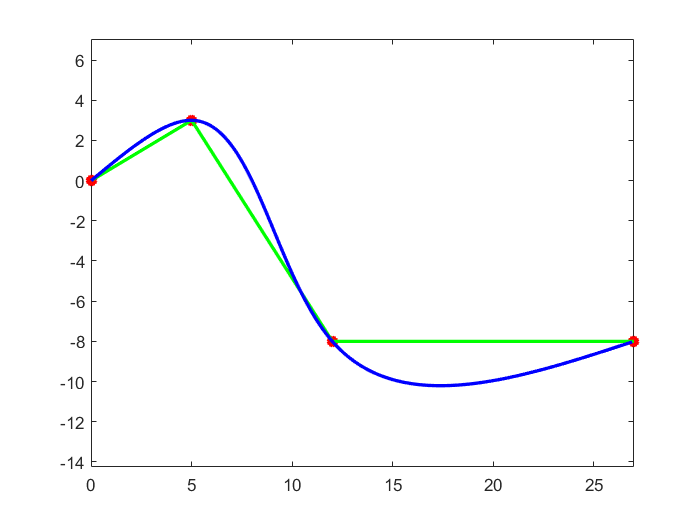23 Jul

#### Cubic Spline Smoother Algorithm

A cubic spline is a spline constructed of piecewise third-order polynomials which pass through a set of n control points. According to , a cubic spline $$S(x)$$ can be defined as: $$S(x)$$ is a cubic polynomial $$S_j(x)$$ on [$$x_j,x_{j+1}$$], for all $$j = 0,1,...,n-1$$.

$$S_j(x) = a_j + b_j(x-x_j) + c_j(x-x_j)^2 + d_j(x-x_j)^3$$

and $$h_j = x_{j+1} - x_j$$, for all $$j =0,1,...,n-1$$

Solve for coefficients $$a_j,b_j,c_j,d_j$$ by:

• $$S_j(x_{j}) = a_j$$
• $$S_{j+1}(x_{j+1}) = a_{j+1} = a_j + b_j h_j + c_j(h_j)^2 + d_j (h_j)^3$$
• $$S^{'}_{j}(x_j) = b_j$$, also $$b_{j+1} = b_j + 2c_j h_j + 3d_j (h_j)^2$$
• $$S^{''}_{j}(x_j) = 2c_j$$, also $$c_{j+1} = c_j + 3d_j h_j$$
• Natural or clamped boundary conditions

Therefore, the coefficient $$a_j,b_j,c_j,d_j$$ are:

• $$a_j = S_j(x_{j})$$
• $$b_j = \frac{a_{j+1}-a_j}{h_j} - \frac{h_j(2c_j+c_{j+1})}{3}$$
• $$c_j$$ can be solved in $$Ac = B$$ format where $$A\in \mathbf{R}^{n\times n}, B \in \mathbf{R}^{n\times 1}$$, since: $$h_{j-1}c_{j-1} + 2(h_{j-1}+h_j)c_j + h_j c_{j+1} = \frac{3}{h_j}(a_{j+1}-a_j) - \frac{3}{h_{j-1}}(a_j - a_{j-1})$$ for all $$j =1,2,...,n-1$$
• $$d_j = \frac{c_{j+1}-c_j}{3h_j}$$Richard H Bartels, John C Beatty, and Brian A Barsky, An introduction to spline for use in computer graphics and geometric modeling, Morgan Kaufmann, 1995